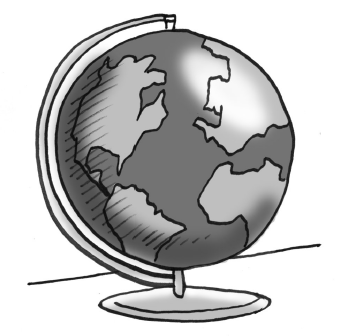### Home > AC > Chapter 9 > Lesson 9.1.1 > Problem9-7

9-7.Solve the problem below by writing and solving an equation. A Guess and Check table may help you write the equation. Be sure to define your variable.

There are a total of $122$ countries in Africa, Europe, and North America (as of 2003). Europe has twice as many countries as North America, and Africa has seven more than Europe. How many countries are in each of these three continents? Write an equation and solve it to answer this question.

Let $n=$ the number of countries in North America.
$2n=$ number of countries in Europe
$2n+7=$ the number of countries in Africa

The total number of countries in all three continents is $122$.

$n+(2n)+(2n+7)=122$

$n=23$
So, there are $23$ countries in North America.
Remember to find the number of countries in Europe and Africa.